# Measuring temporal noise

April 19, 2018
October 30, 2022

Temporal noise is random noise that varies independently from image to image, in contrast to fixed-pattern noise, which remains consistent (but may be difficult to measure because it is usually much weaker than temporal noise). It can be analyzed by Colorcheck and Stepchart and was added to Multicharts and Multitest in Imatest 5.1 (Known as Color/Tone starting in Imatest 5.2)

It can be calculated by two methods.

1. the difference between two identical test chart images (the Imatest recommended method), and

2. the ISO 15739-based method, which where it is calculated from the pixel difference between the average of N identical images (N ≥ 8) and each individual image.

In this post we compare the two methods and show why method 1 is preferred.

(1) Two-image difference method. In any of the modules, read two images. The window shown on the right appears. Select the Read two files for measuring temporal noise radio button.

The two files will be read and their difference (which cancels fixed pattern noise) is taken. Since these images are independent, noise powers add. For indendent images I1 and I2, temporal noise is

$$\displaystyle \sigma_{temporal} = \frac{\sigma(I_1 – I_2)}{\sqrt{2}}$$

In Multicharts and Multitest temporal noise is displayed as dotted lines in Noise analysis plots 1-3 (simple noise, S/N, and SNR (dB)).

(2) Multiple image method. From ISO 15739, sections 6.2.4, 6.2.5, and Appendix A.1.4. Available in  Multicharts and Multitest. Currently we are using simple noise (not yet scene-referred noise). Select between 4 and 16 files. In the multi-image file list window (shown above) select Read n files for temporal noise. Temporal noise is calculated for each pixel j using

$$\displaystyle \sigma_{diff}(j) = \sqrt{ \frac{1}{N} \sum_{i=1}^N (X_{j,i} – X_{AVG,j})^2} = \sqrt{ \frac{1}{N} \sum_{i=1}^N X_{j,i}^2 – \left(\frac{1}{N} \sum_{i=1}^N X_{j,i}\right)^2 }$$

The latter expression is used in the actual calculation since only two arrays, $$\sum X_{j,i} \text{ and } \sum X_{j,i}^2$$, need to be saved. Since N is a relatively small number (between 4 and 16, with 8 recommended), it must be corrected using formulas for sample standard deviation from Identities and mathematical properties in the Wikipedia standard deviation page as well as Equation (13) from ISO 15739.  $$s(X) = \sqrt{\frac{N}{N-1}} \sqrt{E[(X – E(X))^2]}$$.

$$\sigma_{temporal} = \sigma_{diff} \sqrt{\frac{N}{N-1}}$$

We recommend the difference method (1)  when only the magnitude of temporal noise is required. Method (2), which requires many more images (≥ 8 recommended), allows fixed pattern noise and the noise image to be calculated at the same time.

To calculate temporal noise with either method, read the appropriate number of files (2 or ≥4) then push the appropriate radio button on the multi-image settings box.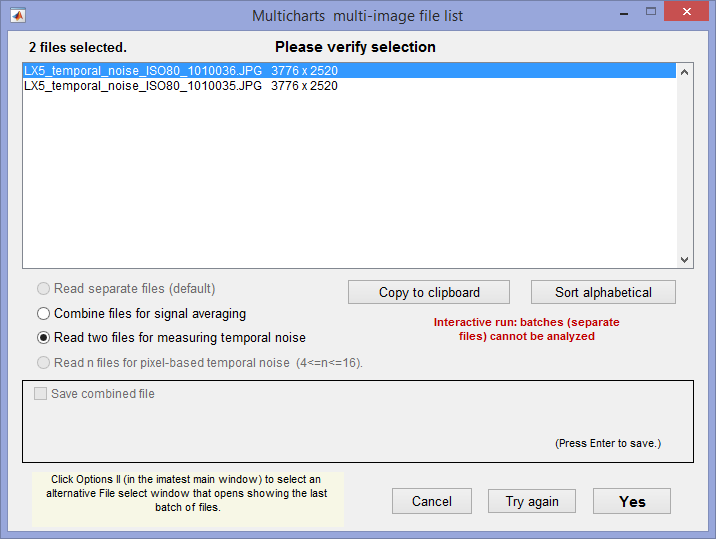Multi-image settings window, showing setting for method 1 (two-image difference).
For ≥4 images (multi-image method), select  • Read n files for … temporal noise, which will be enabled.

### Results for the two methods

The two methods were compared using identical Colorchecker images taken on a Panasonic Lumix LX5 camera (a moderately high quality small-sensor camera now several years old).

#### Difference method (1) (two files)

Here are the Multicharts results for 2 files.Multicharts SNR results for temporal noise, shown as thin dotted lines in the lower plot

#### Multi-file method (2) (4-16 files)

 Here are results (SNR (dB)) for runs with 4, 8, and 16 files. For 4 files, temporal SNR (thin dotted lines) is slightly better than standard noise. Temporal SNR is slightly lower for 8 files and very slightly lower for 16 files. For 8 and 16 files results are closer to the results for 2 files (though differences between 8 and 16 files are very small). The bottom line: We recommend the two-file (difference) method because it is accurate and relatively fast. The multi file method is slower for acquiring and analyzing images— at least 8 images are recommended, so why bother?Temporal SNR from 4 images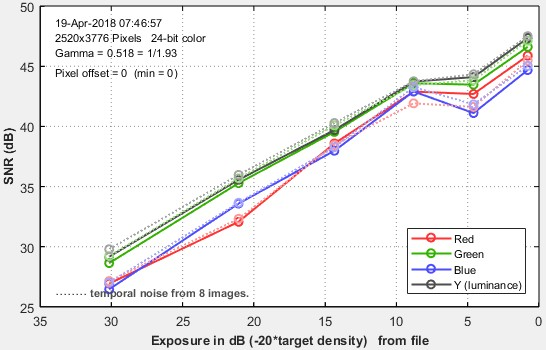Temporal SNR from 8 imagesTempoal SNR from 16 images

### Temporal noise image

 The full Electronic Imaging paper on the noise image can be found on Using images of noise to estimate image processing behavior for image quality evaluation. Noise can be measured anywhere in an image– on edges, etc.– if multiple identical images are acquired. This will lead to some interesting applications.

As we discussed in Flatfield statistics based on EMVA 1288, temporal noise σdiff (j), which is defined for each pixel j, can be displayed as an image. In order for the image to have good enough quality to display, more samples are required than for method (2) (above), which is used to calculate the average temporal noise in a patch — much less demanding than displaying an image. 32 is a reasonable minimum number of samples. 100 or 128 is even better.

Although temporal noise is measured using the same technique as EMVA 1288, there is an important difference. Any arbitrary image (test charts, natural scenes, etc.) can be used; not just flat-field images. This can provide insight into the behavior of image processing over the image — which can be valuable for bilateral filtered images, where the image processing, hence noise, varies over the image surface.

To obtain a temporal noise image, multiple images (typically at least 32) must be signal-averaged. This can be done by combining multiple image files or through direct read (more efficient if it’s available). The method for obtaining noise image is described in detail here. Flatfield Interactive is recommended for displaying temporal noise images. We review the key points.

 For direct data acquisition, make sure the camera and Device Manager are set to correctly capture the image of interest. The Preview has to be turned off to enable the adjustments. Click Save when the image in the Device Manager is correct.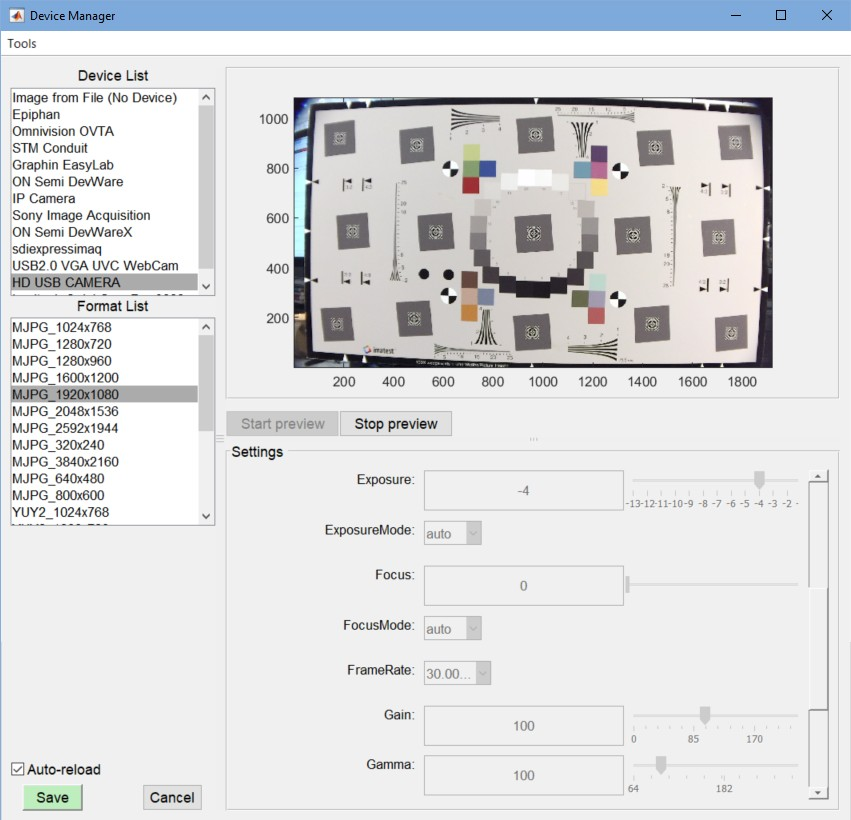In the Flatfield Interactive window, set Signal averaging to a large number (128 reads here), and check Calculate image^2 while averaging. Because of the sequence of operations in Flatfield Interactive, you may need to read an image (before you have the correct settings), then make the settings, then reread. You may want to crop the image when you read it to make it easier to examine specific regions of interest.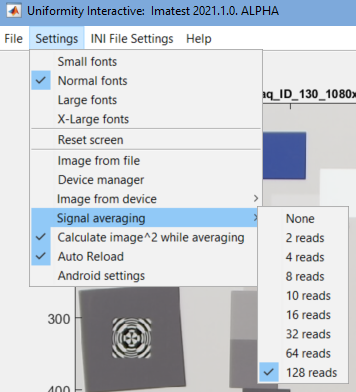Here is the original image (cropped). This image is virtually noiseless because the L = 128 averages increases the SNR (Signal-to-Noise Ratio) by 21 dB (3*log2(L)).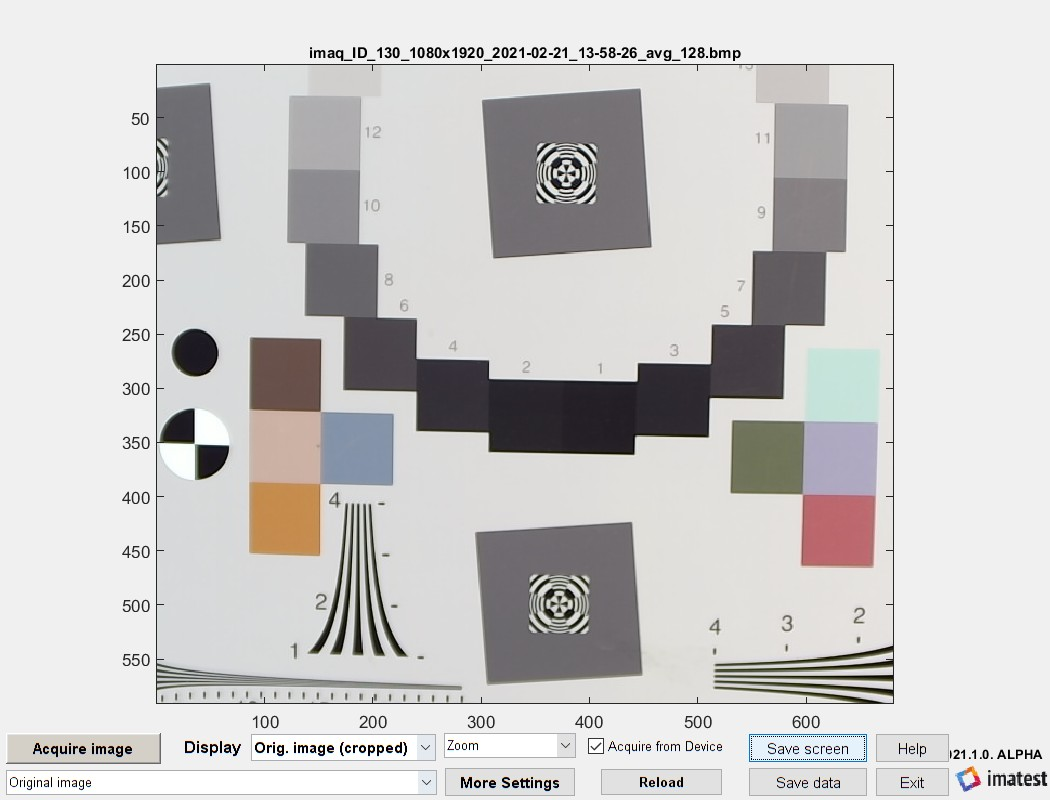Here is the noise image displayed auto-lightened. This gives a good picture of the noise, but lacks quantitative information. As expected, noise is largest near sharp edges and low in smooth areas of the chart. There is no noise in the white part of the registration mark because it’s fully saturated (pure white).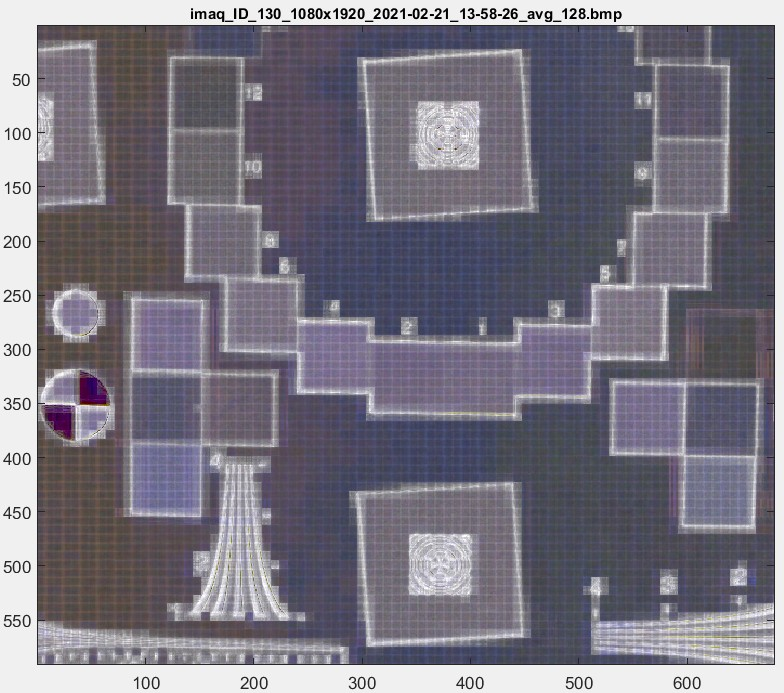Here is the noise image displayed in pseudocolor, which a numeric scale on the right.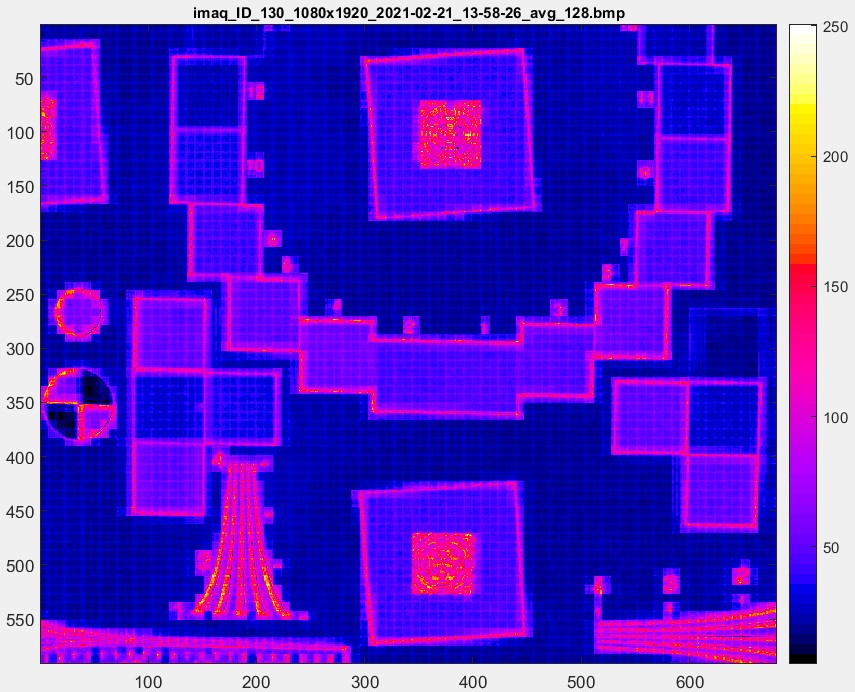Finally, here is the pseudocolor image greatly enlarged. The 8×8 pixel JPEG artifacts, characteristic of medium-low quality JPEG compression, are plainly visible.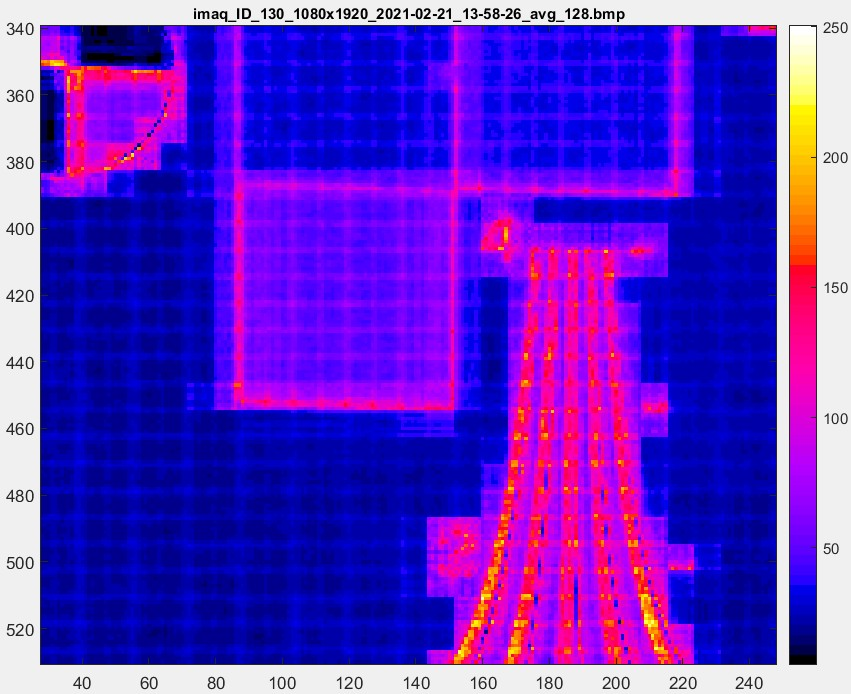## 2 thoughts on “Measuring temporal noise”

1. Hello, I have some questions about the formula of method one.
Is the exact calculation in detail is to calculate the difference between two images in digital level pixel by pixel,
then get the standard deviation of the whole image.
But where is the one over square root of 2 come from?

• When we take the mean of n images, we sum the n images and divide by n to get the mean. We do this because the image signal is correlated from image to image. For finding temporal noise we subtract 2 images. But because noise is UNcorrelated from image to image, noise POWER (voltage^2) ADDS (even when we SUBTRACT two images). The mean noise power is the sum of the noise voltages^2 divided by n (2 in this case). Since noise voltage is the square root of noise power, dividing power by 2 is equivalent to dividing the noise voltage by the square root of 2.

This site uses Akismet to reduce spam. Learn how your comment data is processed.